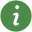# Operators

Operators (+, der, size, ...)# Package ContentsElementaryOperators Elementary operators (+, >, or, ..)'abs()' abs()'acos()' acos()'actualStream()' actualStream()'array()' array()'asin()' asin()'assert()' assert()'atan()' atan()'atan2()' atan2()'cardinality()' cardinality()'cat()' cat()'ceil()' ceil()'change()' change()'connect()' connect()'Connections.branch()' Connections.branch()'Connections.root()' Connections.root()'Connections.potentialRoot()' Connection.potentialRoot()'Connections.isRoot()' Connections.isRoot()'Connections.rooted()' Connections.rooted()'cos()' cos()'cosh()' cosh()'cross()' cross()'delay()' delay()'der()' der()'diagonal()' diagonal()'div()' div()'edge()' edge()'exp()' exp()'fill()' fill()'floor()' floor()'homotopy()' homotopy()'identity()' identity()'initial()' initial()'inStream()' inStream()'Integer()' Integer()'integer()' integer()'linspace()' linspace()'log()' log()'log10()' log10()'matrix()' matrix()'max()' max()'min()' min()'mod()' mod()'ndims()' ndims()'noEvent()' noEvent()'ones()' ones()'outerProduct()' outerProduct()'pre()' pre()'product()' product()'reinit()' reinit()'rem()' rem()'rooted()' rooted() - deprecated'sample()' sample()'scalar()' scalar()'semiLinear()' semiLinear()'sign()' sign()'sin()' sin()'sinh()' sinh()'size()' size()'skew()' skew()'smooth()' smooth()'sqrt()' sqrt()'String()' String()'sum()' sum()'symmetric()' symmetric()'tan()' tan()'tanh()' tanh()'terminal()' terminal()'terminate()' terminate()'transpose()' transpose()'vector()' vector()'zeros()' zeros()

# Information

This information is part of the Modelica Standard Library maintained by the Modelica Association.

In this chapter operators of Modelica are documented. Elementary operators, such as "+" or "-" are overloaded and operate on scalar and array variables. Other operators have the same syntax as a Modelica function call. However, they do not behave as a Modelica function, either because the result depends not only on the input arguments but also on the status of the simulation (such as "pre(..)"), or the function operates on input arguments of different types (such as "String(..)"). Neither of these "functions" can be defined with a "standard" Modelica function and are therefore builtin operators of the Modelica language (with exception of the basic mathematical functions, sin, cos, tan, asin, acos, atan, atan2, sinh, cosh, tanh, exp, log, log10 that are provided for convenience as built-in functions).